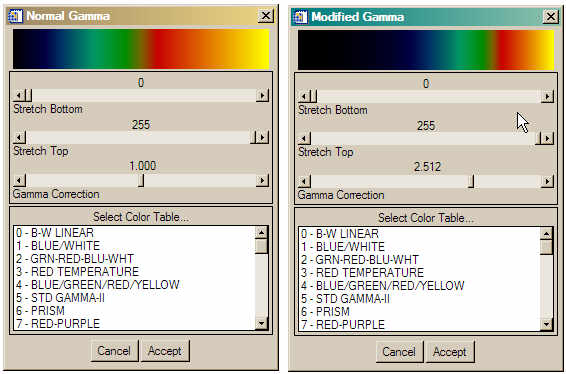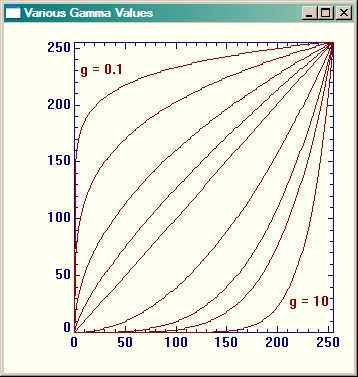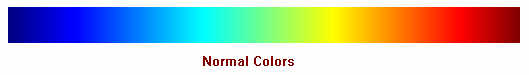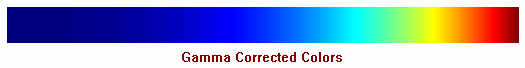Logarithmic Color Table

QUESTION: I know IDL comes with a number of color tables, but they are all scaled linearly. I need a logarithmically scaled color table. How can I create one?ANSWER: The way a color table is scaled is normally determined by the “gamma” of the color table. Normally, the gamma is 1, which results in a linearly scaled color table. But the gamma correction can be changed. If you look, for example, you will find a Gamma Correction slider on XLoadCT or XColors. The slider can be used to change the color look-up ramp to a logarithmic expression.Gamma correction sliders can be found on interactive color tools.

The slider is normally set up so the values of gamma can range between 0.1 and 10.

Let's look at a plot of a selection of a few gamma values.

PRO PlotGamma

; Plot to hold gamma plots.
Plot, Indgen(256), /NoData, XStyle=1, YStyle=1, Font=0, \$
Background=FSC_Color('ivory'), Color=FSC_Color('navy')

; Draw plots with different gammas.
gamma = [0.1, 0.25, 0.5, 0.75, 1.0, 2.0, 3.5, 5.0, 10]
FOR j=0,8 DO BEGIN
d = Findgen(256)^gamma[j]
ramp = Round(d *256 / (Max(d) > 1e-6))
OPlot, ramp, Color=FSC_Color('Dark Red'
ENDFOR

XYOUTS, 0.28, 0.85, /Normal, Alignment=0.5, Font=0, 'g = 0.1'
XYOUTS, 0.87, 0.19, /Normal, Alignment=0.5, Font=0, 'g = 10'

ENDA plot of gamma values ranging from 0.1 to 10.

So, to turn a linear color ramp into a logarithmic color ramp, you have to apply a gamma correction to the linear color vectors. For example, consider the Red-Blue color table, number 33. To load the color table and obtain the color vectors, type this:

IDL> TVLCT, r, g, b, /Get

Next, we have to decide what kind of ramp we want. Suppose we want a ramp with gamma=2.5. We apply the correction to each vector and load the new colors like this:

IDL> gamma = 2.5
IDL> index = Findgen(256)
IDL> distribution = index^gamma > 1e-6
IDL> colorindex = Round(distribution * 255 / (Max(distribution) > 1e-6))
IDL> TVLCT, r[colorindex], g[colorindex], b[colorindex]

You see the result in the figures below.Normal colors verses gamma adjusted colors.Web Coyote's Guide to IDL Programming